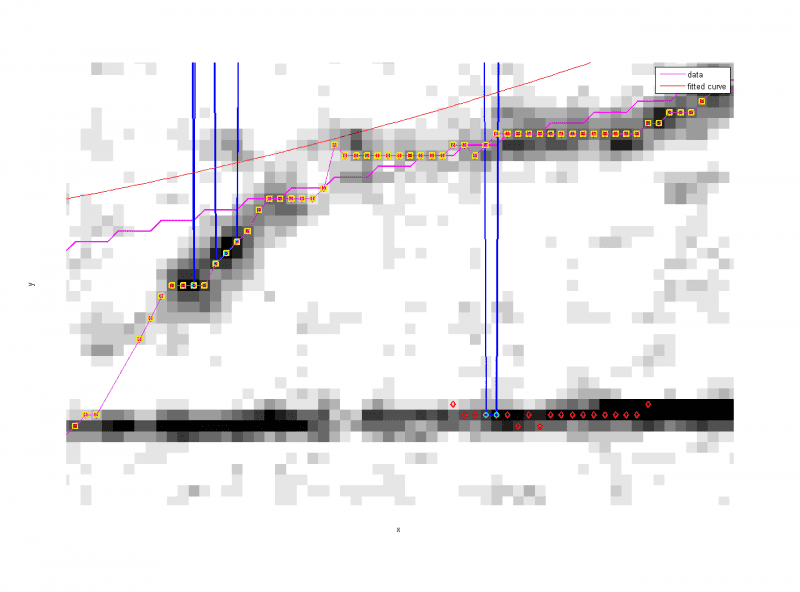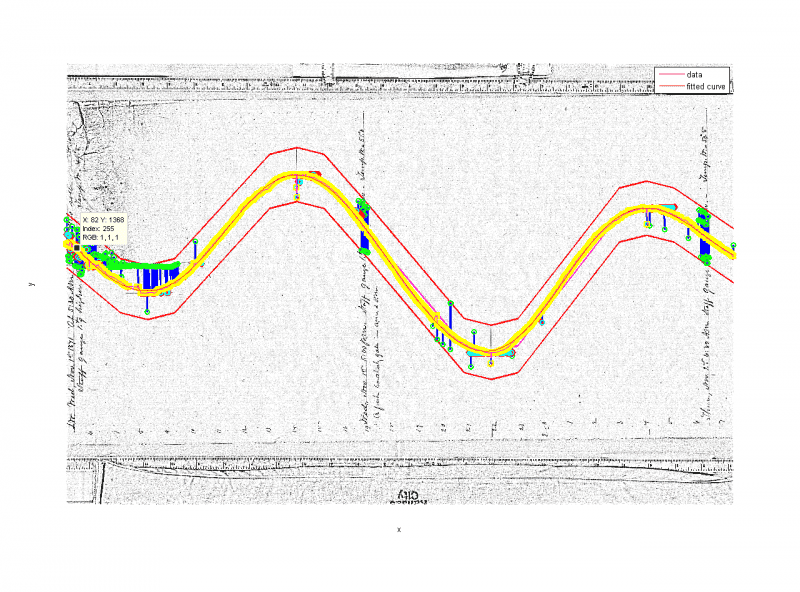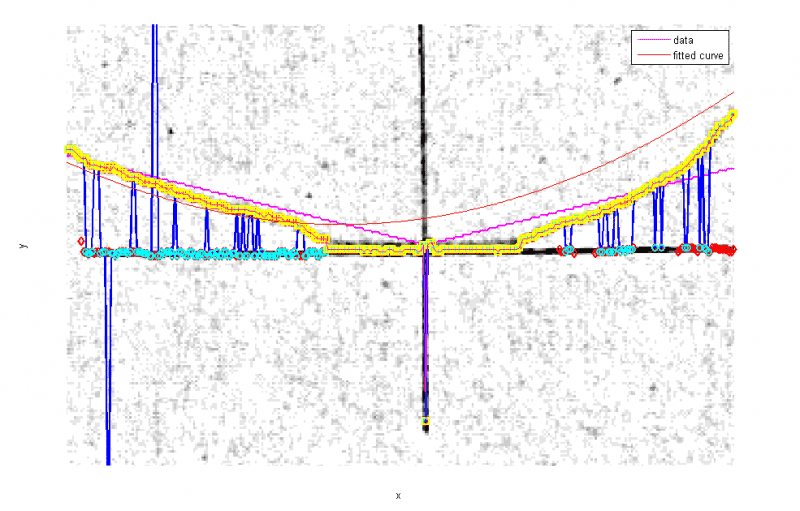# Sinusoidal fit with some points fixed

I'm doing some image processing of some tidal lines that I'm trying to digitize from photographs using Matlab. The digitization is heavily dependent on the indexing of the image of x-values (time) and y-values (water level). After some initial processing based on pixel contrast, line continuity and minimum color (darkest) criteria, I need to fit a sinusoid to the resulting selected index pairs. For every x-value there is only one y-value.

The gist of the digitization procedure is that the contrast values are initially chosen to generate a series of line segments, which are then expanded upon by a forward and backward contrast trace (like the old-school video game Snake). It's an iterative process where some line segments get eliminated from the overall array based on length and RMSE criteria. However, the length criteria can be too restricted and eliminates lines that really are part of the tidal signal but they just don't fully connect to a larger line because of white space existing between the lines.

My problem is that I need to find a way of reintroducing these eliminated 'true' line segments without reintroducing 'false' tidal line segments. The method I came up with is to do a full image-scale sinusoidal curve fit using the fit and fitOptions functions. Then having the segments re-introduce if they intersect that fit line or have a similar slope over the line segment time domain.

This doesn't have to be a fit to determine the tidal constants as with most tidal harmonic analysis. Just accurate enough to get a precise fit. The problem, as with most harmonic analysis, is that the highs and lows are not really accurate. This accuracy is important for the image processing because it forces the ebb and flood regions to be constrained within the time of the extrema.

Is there a way to fix the high and low index values in place while the remaining points are fit to the curve?

Here is the set-up I have now:

xinput( yinput == 9999 ) = [];
yinput( yinput == 9999 ) = [];

% ffit = fittype('sin3');
fitOptions = fitoptions('sin5');
newOptions = fitoptions(fitOptions,'Robust','Bisquare','Normalize','on');
y_fit = fit( xinput, yinput, 'sin5', newOptions );​

I have it at 'sin5' because it increases the accuracy of the fit and not because of the number of potential tidal constituents being 5 (i.e. M2, S1, K1, M4, P1 ).

However, I have also thought about establishing the relative deterministic frequencies of the above mentioned constituents in relation to the pixel span. This might cut down on the number of iterations required. Then again, this might decrease accuracy because the image only shows the tide signal for a day and a half rather than a month necessary to actually do harmonic analysis.

Last edited:

This is the image zoomed in:1. The blue lines are initial contrast points that the segments grow from.
2. The cyan points indicate a segment that was retained by the length and RMSE requirement
3. The red points are the forward and backward pixels from the cyan point that were not chosen to be included because they were further away from the thick magenta line.
4. The yellow points are the final line values for that iteration.
5. The red line is the fitted sinusoid based on 'sin5'
6. (The thin magenta line is the final line values in line form from the fit - not important because it is the same as the yellow points)
Note that the bottom darkened pixels are not the true tidal line but are cross-hairs indicating high or, in this case, low water (low water is to the left of the image).

Here is the same image fully zoomed out:The green points (hard to distinguish with cyan) indicate a segment that was retained by the length but NOT the RMSE requirement.

The following is a zoom in of the second low water:Essentially the thin red line fit is supposed to be an iterative replacement for the thick magenta line. However, as you can see it doesn't do all the well around the high and low water marks that intersect the cross-hairs. This is important for more than just re-introducing the eliminated 'true' line segments.

The magenta line (and hopefully the future red line) is used to determine which sets of red points are closest to the approximate tidal line that become yellow points. Red points further away are disregarded.

There are occasionally situations where this line is too inaccurate to distinguish between the tide and the cross-hairs (and occasionally hand-written notes).